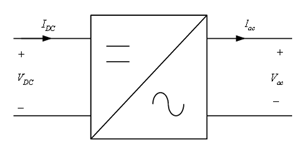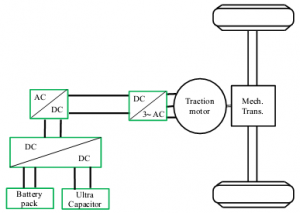Home > dc to ac inverter

# DC-TO-AC INVERTER

A power inverter, or inverter, is an electronic device or circuitry that changes direct currentÂ (DC) to alternating current (AC). A power inverter can be entirely electronic or may be a combination of mechanical effects (such as a rotary apparatus) and electronic circuitry. Static inverters do not use moving parts in the conversion process.

The input voltageÂ , output voltage and frequency, and overall power handling depend on the design of the specific device or circuitry. The inverter does not produce any power; the power is provided by the DC source. Figure below shows Basic DC-AC Inverter Block Diagram.A typical power inverter device or circuit requires a relatively stable DC power source capable of supplying enough current for the intended power demands of the system. The input voltage depends on the design and purpose of the inverter. Figure below shows the Configuration of Electric Vehicle.In figure above it can be seen that the traction motor requires AC input. The main source of electrical power is the battery which is a DC source. The DC output of the battery is bucked or boosted according to the requirement and then converted into AC using a DC-AC inverter. The function of an inverter is to change a dc input voltage to a symmetric ac output voltage of desired magnitude and frequency. The output voltage waveforms of ideal inverters should be sinusoidal. However, the waveforms of practical inverters are non-sinusoidal and contain certain harmonics.